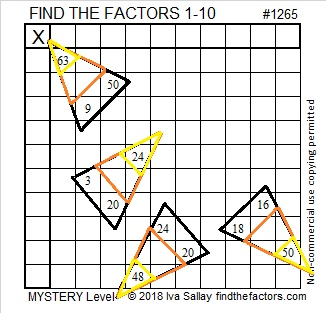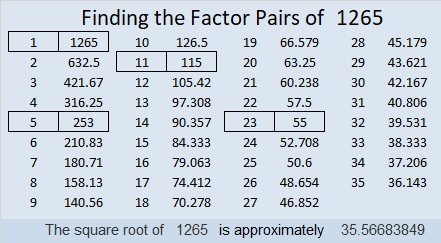# 1265 More Candy Corn

People don’t each candy corn every day and the only corn in it is corn syrup.  We usually only see it this time of year. Here’s a puzzle with some candy corn for you to enjoy:Print the puzzles or type the solution in this excel file: 10-factors-1259-1270

• 1265 is a composite number.
• Prime factorization: 1265 = 5 × 11 × 23
• The exponents in the prime factorization are 1, 1, and 1. Adding one to each and multiplying we get (1 + 1)(1 + 1)(1 + 1) = 2 × 2 × 2 = 8. Therefore 1265 has exactly 8 factors.
• Factors of 1265: 1, 5, 11, 23, 55, 115, 253, 1265
• Factor pairs: 1265 = 1 × 1265, 5 × 253, 11 × 115, or 23 × 55
• 1265 has no square factors that allow its square root to be simplified. √1265 ≈ 35.566841265 is the sum of the fifteen prime numbers from 53 to 113.

1265 is the hypotenuse of a Pythagorean triple:
759-1012-1265 which is (3-4-5) times 253

This site uses Akismet to reduce spam. Learn how your comment data is processed.